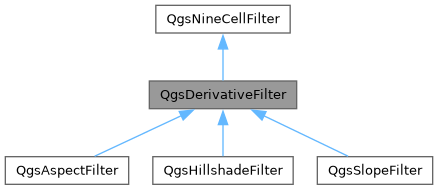QGIS API Documentation 3.33.0-Master (bf22a165b3)
Searching...
No Matches
QgsDerivativeFilter Class Referenceabstract

Adds the ability to calculate derivatives in x- and y-directions. More...

`#include <qgsderivativefilter.h>`

Inheritance diagram for QgsDerivativeFilter:[legend]

## Public Member Functions

QgsDerivativeFilter (const QString &inputFile, const QString &outputFile, const QString &outputFormat)

float processNineCellWindow (float *x11, float *x21, float *x31, float *x12, float *x22, float *x32, float *x13, float *x23, float *x33) override=0
Calculates output value from nine input values.Public Member Functions inherited from QgsNineCellFilter
QgsNineCellFilter (const QString &inputFile, const QString &outputFile, const QString &outputFormat)
Constructor that takes input file, output file and output format (GDAL string)

virtual ~QgsNineCellFilter ()=default

double cellSizeX () const

double cellSizeY () const

double inputNodataValue () const

double outputNodataValue () const

int processRaster (QgsFeedback *feedback=nullptr)
Starts the calculation, reads from mInputFile and stores the result in mOutputFile.

void setCellSizeX (double size)

void setCellSizeY (double size)

void setInputNodataValue (double value)

void setOutputNodataValue (double value)

void setZFactor (double factor)

double zFactor () const

## Protected Member Functions

float calcFirstDerX (float *x11, float *x21, float *x31, float *x12, float *x22, float *x32, float *x13, float *x23, float *x33) const
Calculates the first order derivative in x-direction according to Horn (1981)

float calcFirstDerY (float *x11, float *x21, float *x31, float *x12, float *x22, float *x32, float *x13, float *x23, float *x33) const
Calculates the first order derivative in y-direction according to Horn (1981)Protected Attributes inherited from QgsNineCellFilter
double mCellSizeX = -1.0

double mCellSizeY = -1.0

QString mInputFile

float mInputNodataValue = -1.0
The nodata value of the input layer.

QString mOutputFile

QString mOutputFormat

float mOutputNodataValue = -1.0
The nodata value of the output layer.

double mZFactor = 1.0
Scale factor for z-value if x-/y- units are different to z-units (111120 for degree->meters and 370400 for degree->feet)

## Detailed Description

Adds the ability to calculate derivatives in x- and y-directions.

Needs to be subclassed (e.g. for slope and aspect).

Definition at line 30 of file qgsderivativefilter.h.

## ◆ QgsDerivativeFilter()

 QgsDerivativeFilter::QgsDerivativeFilter ( const QString & inputFile, const QString & outputFile, const QString & outputFormat )

Definition at line 20 of file qgsderivativefilter.cpp.

## ◆ calcFirstDerX()

 float QgsDerivativeFilter::calcFirstDerX ( float * x11, float * x21, float * x31, float * x12, float * x22, float * x32, float * x13, float * x23, float * x33 ) const
protected

Calculates the first order derivative in x-direction according to Horn (1981)

Definition at line 26 of file qgsderivativefilter.cpp.

## ◆ calcFirstDerY()

 float QgsDerivativeFilter::calcFirstDerY ( float * x11, float * x21, float * x31, float * x12, float * x22, float * x32, float * x13, float * x23, float * x33 ) const
protected

Calculates the first order derivative in y-direction according to Horn (1981)

Definition at line 93 of file qgsderivativefilter.cpp.

## ◆ processNineCellWindow()

 float QgsDerivativeFilter::processNineCellWindow ( float * x11, float * x21, float * x31, float * x12, float * x22, float * x32, float * x13, float * x23, float * x33 )
overridepure virtual

Calculates output value from nine input values.

The input values and the output value can be equal to the nodata value if not present or outside of the border. Must be implemented by subclasses.

First index of the input cell is the row, second index is the column

Parameters
 x11 surrounding cell top left x21 surrounding cell central left x31 surrounding cell bottom left x12 surrounding cell top central x22 the central cell for which the value will be calculated x32 surrounding cell bottom central x13 surrounding cell top right x23 surrounding cell central right x33 surrounding cell bottom right
Returns
the calculated cell value for the central cell x22

Implements QgsNineCellFilter.

Implemented in QgsAspectFilter, QgsHillshadeFilter, and QgsSlopeFilter.

The documentation for this class was generated from the following files: